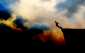# Help please: $(1+e^x y+x e^x y) dx + (x e^x + 2) dy=0$

1 post / 0 new
Joshua Melegrit...Help please: $(1+e^x y+x e^x y) dx + (x e^x + 2) dy=0$

(1+e^(x) y+x e^(x) y) dx + (x e^(x) + 2) dy=0

• Mathematics inside the configured delimiters is rendered by MathJax. The default math delimiters are $$...$$ and $...$ for displayed mathematics, and $...$ and $...$ for in-line mathematics.